# Linear Simulations: We simulate ITG with adiabatic electrons. The normalized ion temperature gradient is                    and the density gradient is                    . The comparison for the growth rates and frequencies is shown below:# Next, we show ITG with kinetic electrons, the gradients remain as before, and in addition we set                      and The comparison for the growth rates and frequencies is shown below: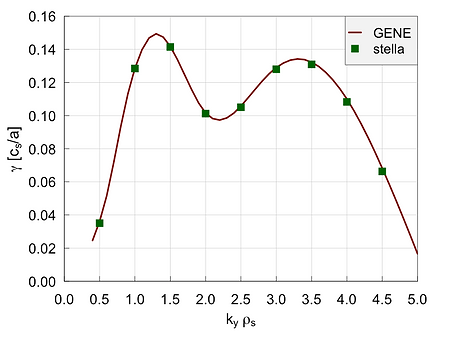# Zonal flow response: We use                   . The comparison for the squared zonal potential is shown below: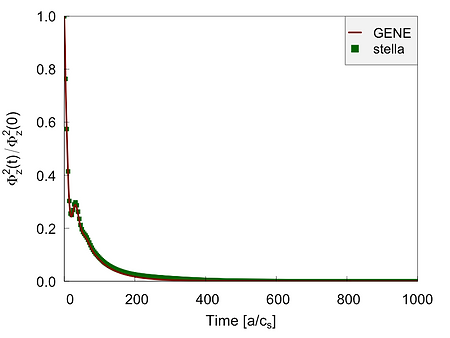# Nonlinear Simulations: We simulate ITG with adiabatic electrons. The normalized ion temperature gradient is                  and the density gradient is                    . The comparison for the ion heat fluxes rates is shown below:# In addition, we show the comparison for the particle flux:# Testing the parallel b.c. We present the comparison for ITG turbulence simulations for stella, using either the twist and shift boundary condition or the periodic boundary condition.# Relating ITG heat flux with max-J. In the following stella simulations we apply                    plus a density gradient. We use the vacuum W7-X configurations: ref_128 (mr=8%) and ref_404 (mr=-4%), also a tokamak as reference case. The surface for the simulations is r/a=0.75.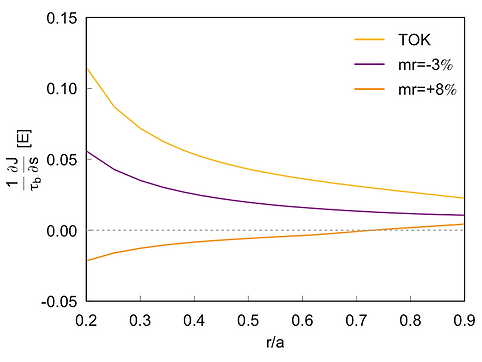# Formally, only W7-X mr=8% is max-J. However, we may characterize the configurations according to a degree of max-J. For instance, mr=8% has a higher degree than mr=-4%, which in turn has a higher degree than TOK. We should then expect that the ITG ion heat fluxes respond to the density gradient according to that degree: for TOK, the ion heat flux is enhanced by the density gradient, for mr=-4% the heat flux is almost unchanged, and for mr=8% the ion heat flux is reduced.# mr=+8%# mr=-4%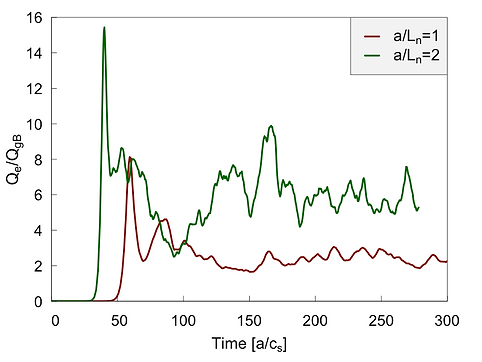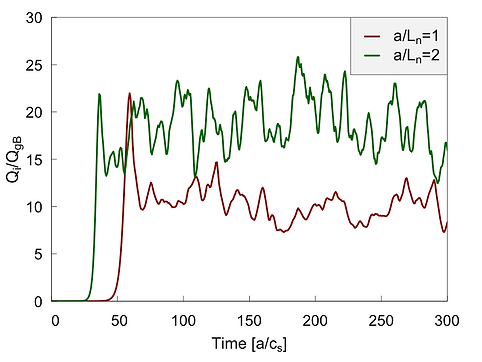# TOK# Next, we show ITG with kinetic electrons, the gradients remain as before, and in addition we set                      and The comparison for ion and electron heat fluxes is shown below: